Search

Graphing Rational Functions:
Another Example
(page 3 of 4)

Sections: Introduction, Examples, The special case with the "hole"• Graph the following:
•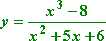First I'll find any vertical asymptotes, by setting the denominator equal to zero and solving:

x2 + 5x + 6 = 0
(x + 3)(x + 2) = 0

x = 3, x = 2

 So I've got two vertical asymptotes: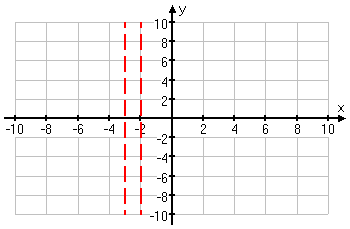Now I'll look for the horizontal or slant asymptote. The numerator is a cubic (that is, it has degree three) and the denominator is a quadratic (that is, it has degree two). Since the numerator is of greater degree, then this graph has a slant asymptote. I'll use long division to find the equation of the slant asymptote:   Copyright © Elizabeth Stapel 2003-2011 All Rights Reserved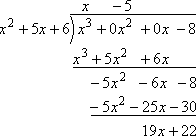Then the slant asymptote is the line y = x  5. I'll dash this in: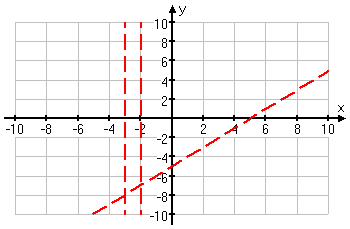Now I'll find any intercepts, by plugging zero in for each variable, in turn:

x = 0:  y = (0  8)/(0 + 0 + 6) = 4/3

y = 0:  0 = (x3  8) / (x2 + 5x + 6)

0 = x3  8 = (x  2)(x2 + 2x + 4)

0 = x  2

2 = x

I noted that the x2 + 2x + 4 factor has no solutions, so I couldn't have gotten any x-intercepts from it.

 My intercepts are at (0, 4/3) and (2, 0).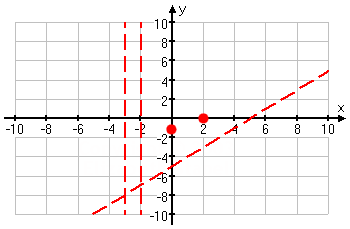This still leaves a lot of the graph unaccounted for. In order to be sure of what is going on here, I'll plot quite a few more points.

 x y 8 17.333 5 22.167 4 36 3.5 67.833 2.9 359.878 2.5 94.5 2.4 90.933

 x y 2.1 191.789 1.9 135.082 1.5 15.167 1 4.5 4 1.333 7 3.722 10 6.359

Hmm... Some of those y-values are pretty darned huge....

 I think I'll need to redraw my axes just a smidge, to account for those very large y-values; then I'll plot my points.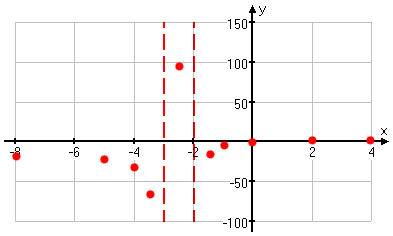Now that I have a good feel for what the graph is doing, I'll draw it in: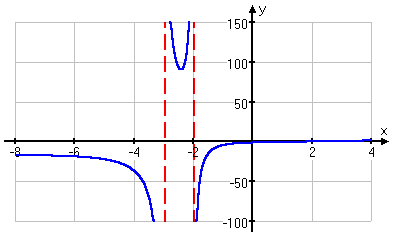It used to be highly unusual for a graph to have such large y-values, but now that many students are trying to get by with just copying the pretty pictures from their graphing calculators, exercises are being written with graphing calculators in mind. Much of the above graph (especially the middle part) would not show up on the graphing calculator, so a lazy student would be "caught" when he didn't include that middle part in his graph.

For this reason, you shouldn't be surprised occasionally to encounter graphs with very large y-values. When this happens, just adjust your axis scales. I left the x-axis scale fairly small (counting by 2's), but changed the y-axis scale to something large (counting by 50's). Don't think that the axis scales always have to be the same, or that you always have to count by 1's. Be prepared to be flexible.

<< Previous  Top  |  1 | 2 | 3 | 4  |  Return to Index  Next >>

 Cite this article as: Stapel, Elizabeth. "Graphing Rational Functions: Another Example." Purplemath. Available from     https://www.purplemath.com/modules/grphrtnl3.htm. Accessed [Date] [Month] 2016

Reviews of
Internet Sites:
Free Help
Practice
Et Cetera

Study Skills Survey

Tutoring from Purplemath
Find a local math tutor